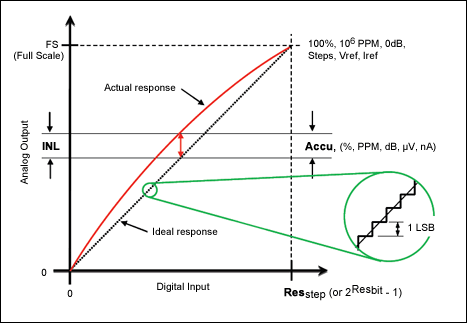### Abstract

The Accuracy Calculator (ACCU) aids in the design and analysis of data-converter application circuits. It calculates the DC accuracy of an ideal data converter. The program is for use with a HP® 50g calculator or free PC emulator.

### Introduction

Steve Edwards, an experienced analog design engineer, has written several calculators to automate repetitive tasks. These tools are being shared to help other analog design engineers who select, specify, and characterize analog circuits. We will summarize the functionality of one such tool, the Accuracy Calculator.

### Accuracy Calculator

The Accuracy Calculator (ACCU) aids in the design and analysis of data-converter application circuits. It calculates the DC accuracy of an ideal data converter, covering both analog-to-digital converters (ADCs) and digital-to-analog converters (DACs). The DC accuracy of a data converter is the measure of the maximum deviation from the ideal linear transfer function. The HP 50g handheld calculator is a convenient tool, but there is also a free emulator that runs on many computers that use the Windows® operating system.The complete package of information for the accuracy calculator, including the free emulator, can be found at Steve's Analog Design Calculators page.

The AACU User's Guide (available as part of the AACU program package) provides instructions for the calculator's use, the theory and equations behind the data converter accuracy issue, and most importantly, a practical example of its use in circuit design and analysis.

The industry can interchangeably specify a common parameter in different units. The calculator allows any unit to be used for entry, and then displays the alternative units. This allows the user to quickly evaluate devices using different units. For example, resolution may be entered as bits or as a number of steps. Accuracy can be measured in percentage of full scale (%), parts per million (PPM), decibels (dB), or microvolts (µV). Current-based converters are also supported.The user's guide tutorial details how data-converter DC accuracy is calculated. A practical example illustrates how to use the ACCU to solve a common design problem. The example takes us from entering the data, through solving and finding a converter, and provides quick ways to explore alternative accuracy parts.